# RD Sharma Solutions for Class 10 Maths Chapter 16 Surface Areas And Volumes Exercise 16.3

A frustum is a part of a right circular cone when it’s cut by a plane parallel to the base of the cone. Problems on finding the volume and surface area of a frustum of a right circular cone are in this exercise. Students can access the RD Sharma Solutions Class 10 which is prepared by experts at BYJU’S for the sole purpose of strengthening the concepts and problem-solving abilities. Students can also download the RD Sharma Solutions for Class 10 Maths Chapter 16 Surface Areas And Volumes Exercise 16.3 PDF provided below.

## RD Sharma Solutions for Class 10 Maths Chapter 16 Surface Areas And Volumes Exercise 16.3 Download PDF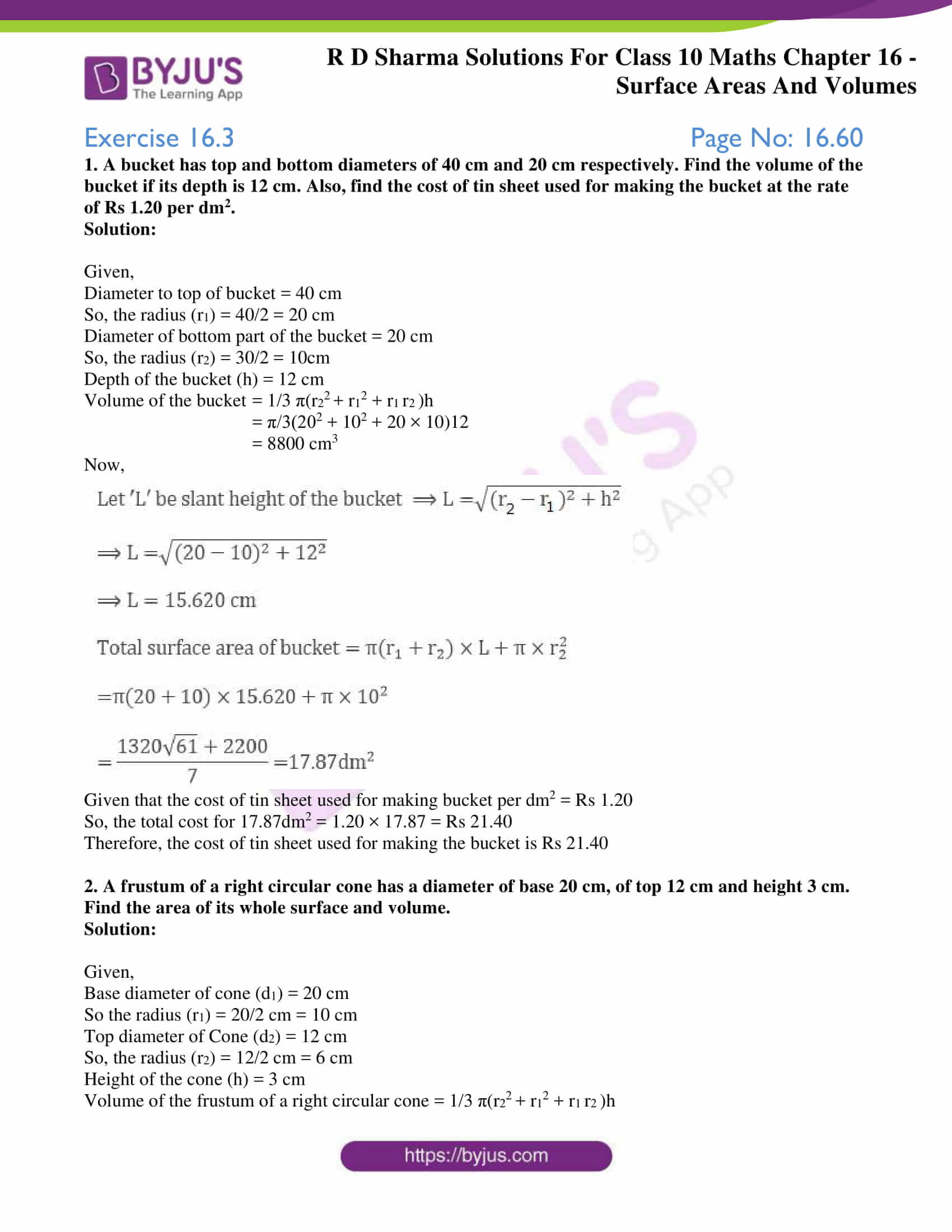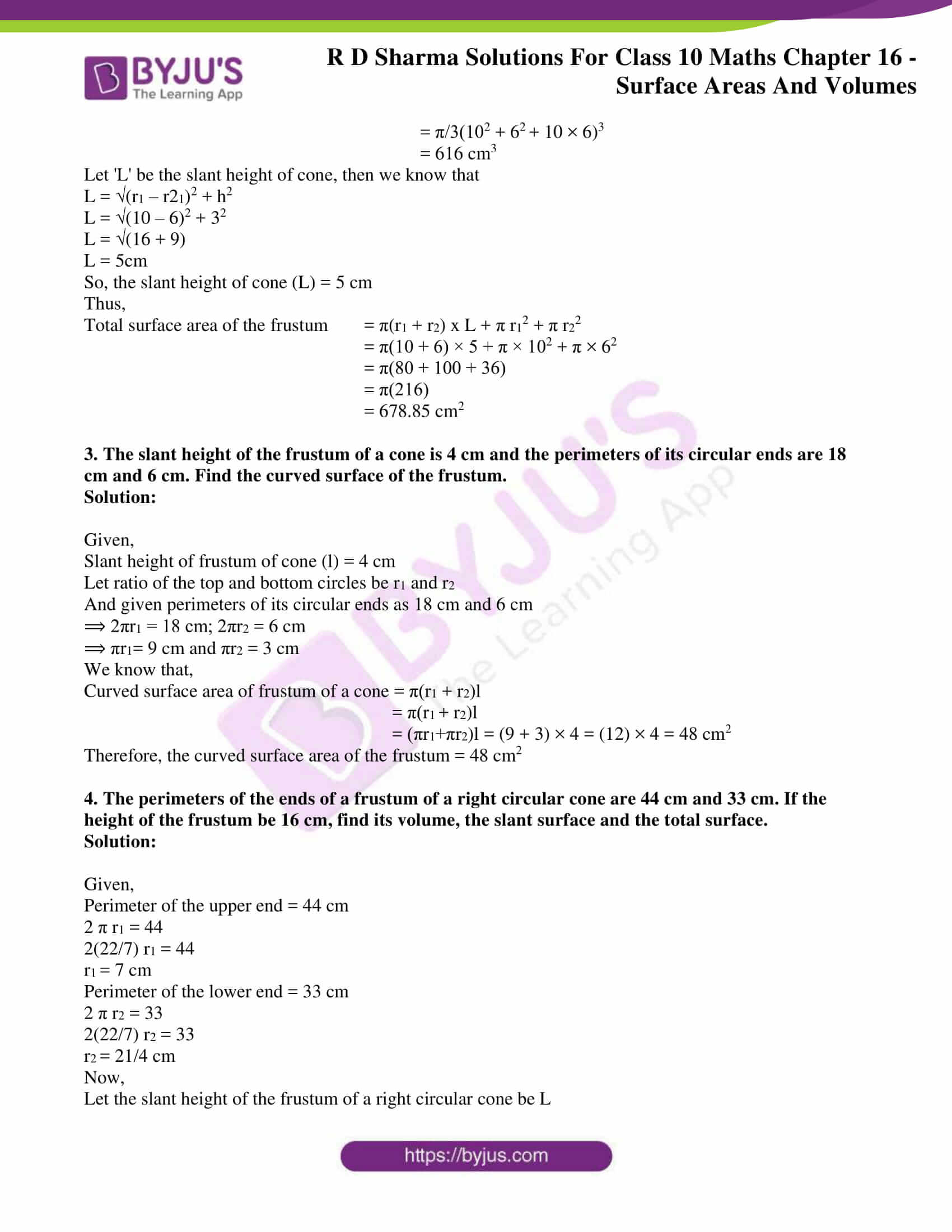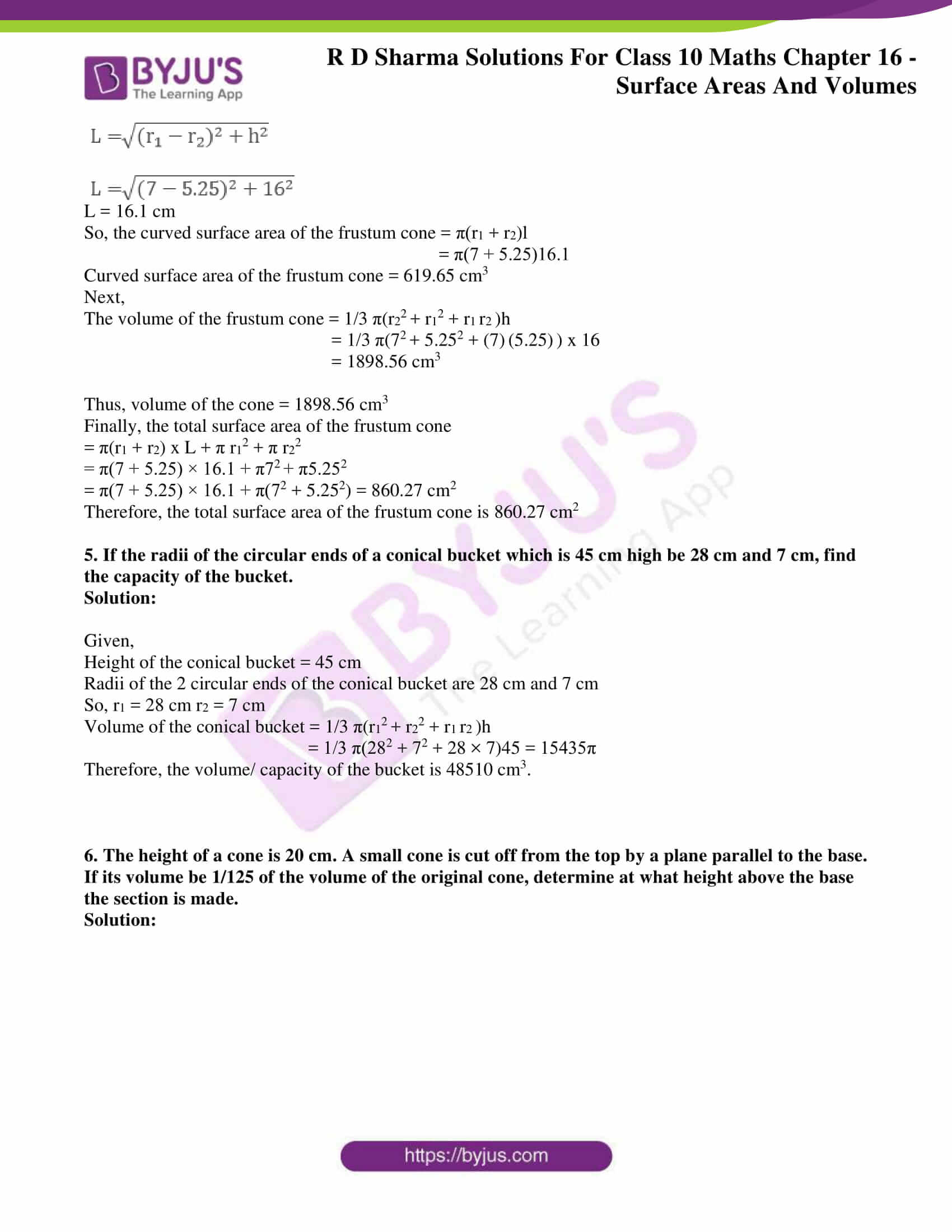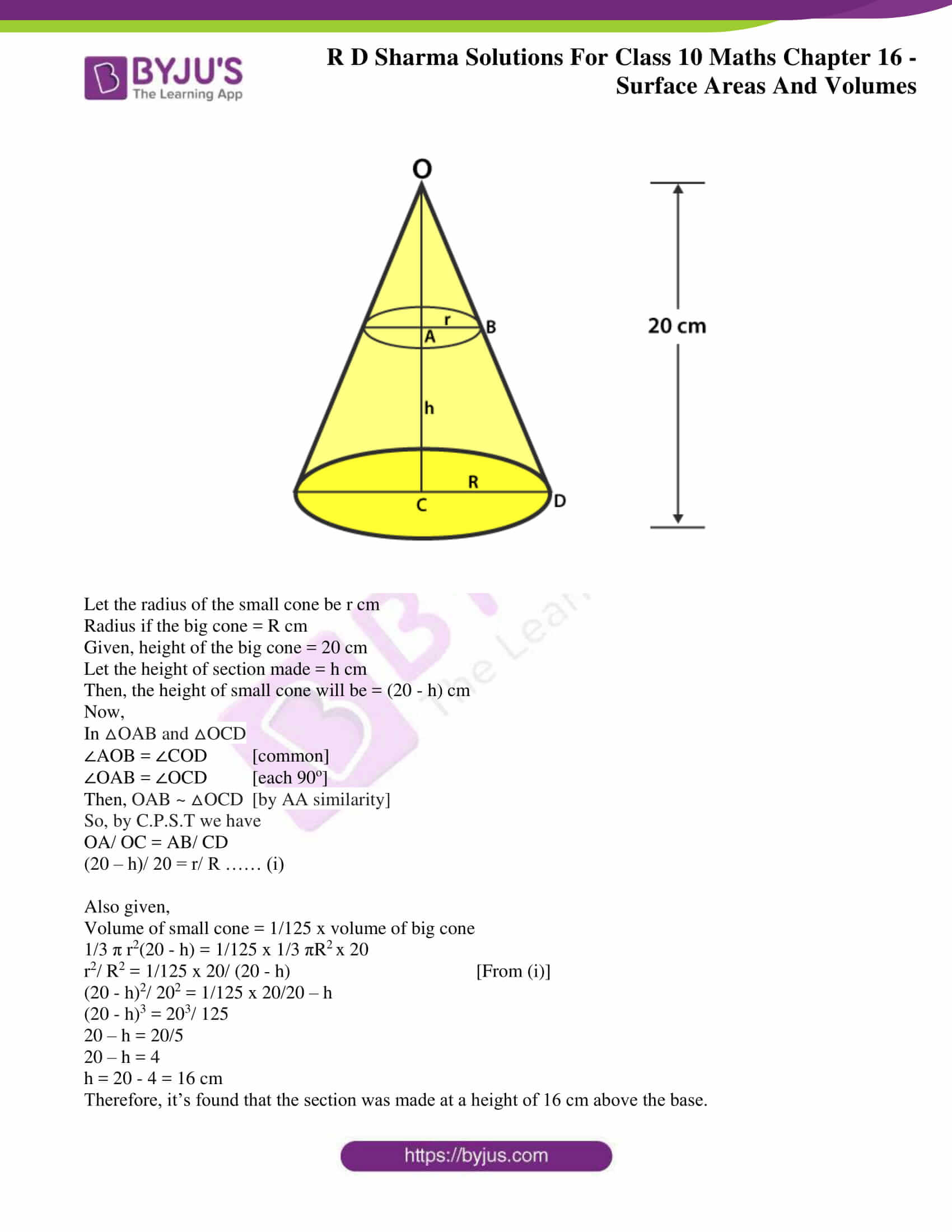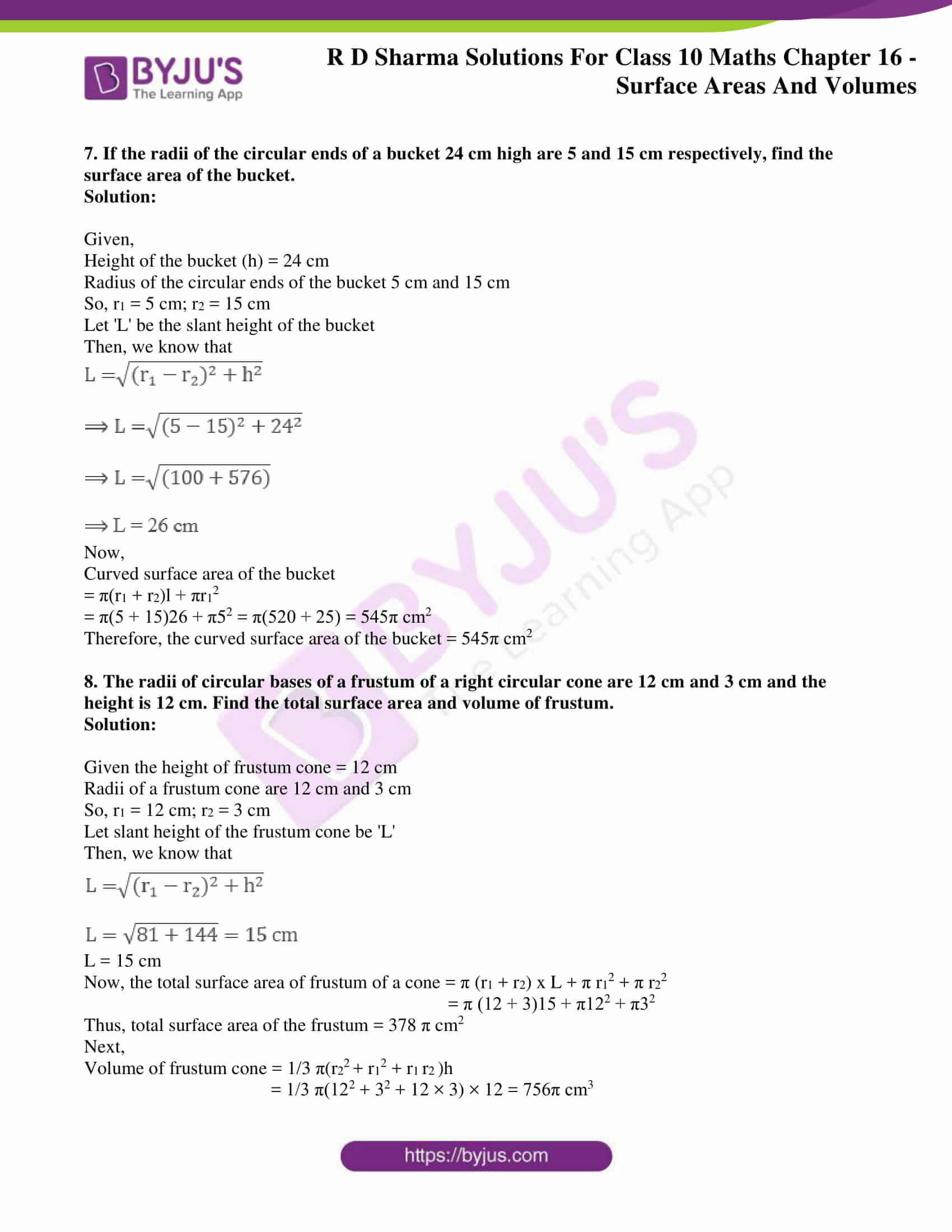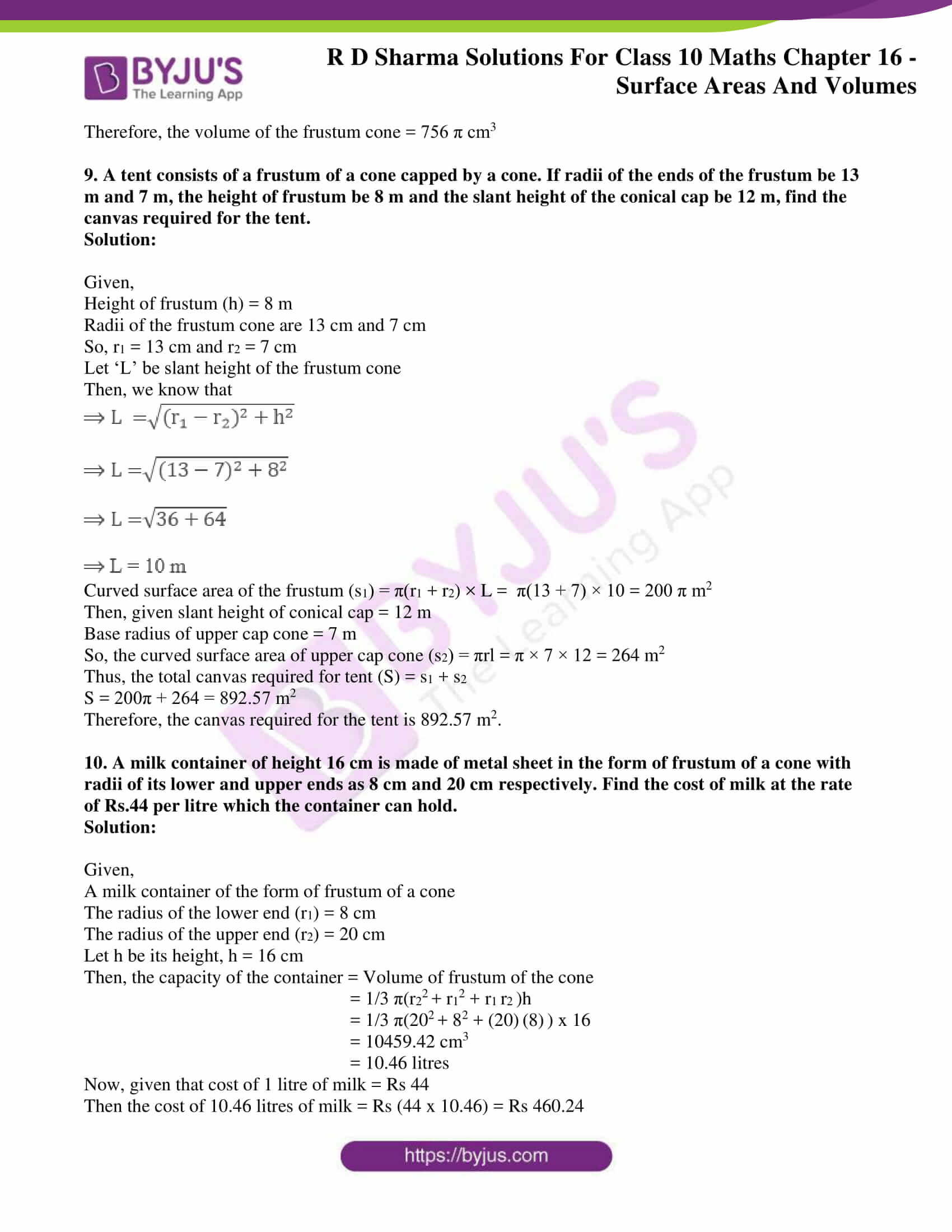### Access RD Sharma Solutions for Class 10 Maths Chapter 16 Surface Areas And Volumes Exercise 16.3

1. A bucket has top and bottom diameters of 40 cm and 20 cm respectively. Find the volume of the bucket if its depth is 12 cm. Also, find the cost of tin sheet used for making the bucket at the rate of Rs 1.20 per dm2.

Solution:

Given,

Diameter to top of bucket = 40 cm

So, the radius (r1) = 40/2 = 20 cm

Diameter of bottom part of the bucket = 20 cm

So, the radius (r2) = 30/2 = 10cm

Depth of the bucket (h) = 12 cm

Volume of the bucket = 1/3 π(r22 + r12 + r1 r)h

= π/3(202 + 102 + 20 × 10)12

= 8800 cm3

Now,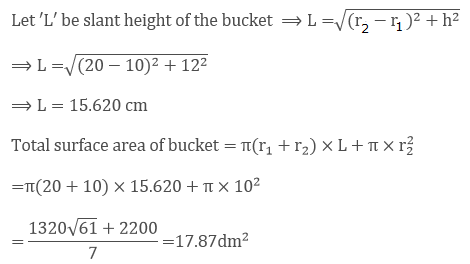Given that the cost of tin sheet used for making bucket per dm2 = Rs 1.20

So, the total cost for 17.87dm2 = 1.20 × 17.87 = Rs 21.40

Therefore, the cost of tin sheet used for making the bucket is Rs 21.40

2. A frustum of a right circular cone has a diameter of base 20 cm, of top 12 cm and height 3 cm. Find the area of its whole surface and volume.

Solution:

Given,

Base diameter of cone (d1) = 20 cm

So the radius (r1) = 20/2 cm = 10 cm

Top diameter of Cone (d2) = 12 cm

So, the radius (r2) = 12/2 cm = 6 cm

Height of the cone (h) = 3 cm

Volume of the frustum of a right circular cone = 1/3 π(r22 + r12 + r1 r)h

= π/3(102 + 6+ 10 × 6)3

= 616 cm3

Let ‘L’ be the slant height of cone, then we know that

L = √(r1 – r21)2 + h2

L = √(10 – 6)2 + 32

L = √(16 + 9)

L = 5cm

So, the slant height of cone (L) = 5 cm

Thus,

Total surface area of the frustum = π(r1 + r2) x L + π r12 + π r22

= π(10 + 6) × 5 + π × 102 + π × 62

= π(80 + 100 + 36)

= π(216)

= 678.85 cm2

3. The slant height of the frustum of a cone is 4 cm and the perimeters of its circular ends are 18 cm and 6 cm. Find the curved surface of the frustum.

Solution:

Given,

Slant height of frustum of cone (l) = 4 cm

Let ratio of the top and bottom circles be r1 and r2

And given perimeters of its circular ends as 18 cm and 6 cm

⟹ 2πr1 = 18 cm; 2πr2 = 6 cm

⟹ πr1= 9 cm and πr2 = 3 cm

We know that,

Curved surface area of frustum of a cone = π(r1 + r2)l

= π(r+ r2)l

= (πr1+πr2)l = (9 + 3) × 4 = (12) × 4 = 48 cm2

Therefore, the curved surface area of the frustum = 48 cm2

4. The perimeters of the ends of a frustum of a right circular cone are 44 cm and 33 cm. If the height of the frustum be 16 cm, find its volume, the slant surface and the total surface.

Solution:

Given,

Perimeter of the upper end = 44 cm

2 π r1 = 44

2(22/7) r1 = 44

r1 = 7 cm

Perimeter of the lower end = 33 cm

2 π r2 = 33

2(22/7) r2 = 33

r2 = 21/4 cm

Now,

Let the slant height of the frustum of a right circular cone be L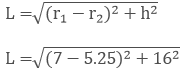L = 16.1 cm

So, the curved surface area of the frustum cone = π(r1 + r2)l

= π(7 + 5.25)16.1

Curved surface area of the frustum cone = 619.65 cm3

Next,

The volume of the frustum cone = 1/3 π(r22 + r12 + r1 r)h

= 1/3 π(72 + 5.252 + (7) (5.25) ) x 16

= 1898.56 cm3

Thus, volume of the cone = 1898.56 cm3

Finally, the total surface area of the frustum cone

= π(r1 + r2) x L + π r12 + π r22

= π(7 + 5.25) × 16.1 + π7+ π5.252

= π(7 + 5.25) × 16.1 + π(72 + 5.252) = 860.27 cm2

Therefore, the total surface area of the frustum cone is 860.27 cm2

5. If the radii of the circular ends of a conical bucket which is 45 cm high be 28 cm and 7 cm, find the capacity of the bucket.

Solution:

Given,

Height of the conical bucket = 45 cm

Radii of the 2 circular ends of the conical bucket are 28 cm and 7 cm

So, r1 = 28 cm r2 = 7 cm

Volume of the conical bucket = 1/3 π(r12 + r22 + r1 r)h

= 1/3 π(282 + 72 + 28 × 7)45 = 15435π

Therefore, the volume/ capacity of the bucket is 48510 cm3.

6. The height of a cone is 20 cm. A small cone is cut off from the top by a plane parallel to the base. If its volume be 1/125 of the volume of the original cone, determine at what height above the base the section is made.

Solution: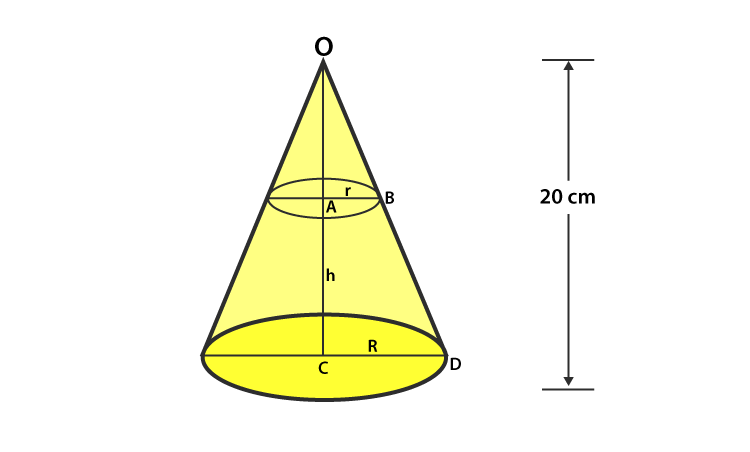Let the radius of the small cone be r cm

Radius if the big cone = R cm

Given, height of the big cone = 20 cm

Let the height of section made = h cm

Then, the height of small cone will be = (20 – h) cm

Now,

In △OAB and △OCD

∠AOB = ∠COD [common]

∠OAB = ∠OCD [each 90o]

Then, OAB ~ △OCD [by AA similarity]

So, by C.P.S.T we have

OA/ OC = AB/ CD

(20 – h)/ 20 = r/ R …… (i)

Also given,

Volume of small cone = 1/125 x volume of big cone

1/3 π r2(20 – h) = 1/125 x 1/3 πR2 x 20

r2/ R2 = 1/125 x 20/ (20 – h) [From (i)]

(20 – h)2/ 202 = 1/125 x 20/20 – h

(20 – h)3 = 203/ 125

20 – h = 20/5

20 – h = 4

h = 20 – 4 = 16 cm

Therefore, it’s found that the section was made at a height of 16 cm above the base.

7. If the radii of the circular ends of a bucket 24 cm high are 5 and 15 cm respectively, find the surface area of the bucket.

Solution:

Given,

Height of the bucket (h) = 24 cm

Radius of the circular ends of the bucket 5 cm and 15 cm

So, r1 = 5 cm; r2 = 15 cm

Let ‘L’ be the slant height of the bucket

Then, we know that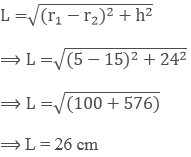Now,

Curved surface area of the bucket

= π(r1 + r2)l + πr12

= π(5 + 15)26 + π52 = π(520 + 25) = 545π cm2

Therefore, the curved surface area of the bucket = 545π cm2

8. The radii of circular bases of a frustum of a right circular cone are 12 cm and 3 cm and the height is 12 cm. Find the total surface area and volume of frustum.

Solution:

Given the height of frustum cone = 12 cm

Radii of a frustum cone are 12 cm and 3 cm

So, r1 = 12 cm; r2 = 3 cm

Let slant height of the frustum cone be ‘L’

Then, we know that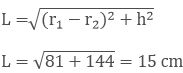L = 15 cm

Now, the total surface area of frustum of a cone = π (r1 + r2) x L + π r12 + π r22

= π (12 + 3)15 + π122 + π32

Thus, total surface area of the frustum = 378 π cm2

Next,

Volume of frustum cone = 1/3 π(r22 + r12 + r1 r)h

= 1/3 π(122 + 32 + 12 × 3) × 12 = 756π cm3

Therefore, the volume of the frustum cone = 756 π cm3

9. A tent consists of a frustum of a cone capped by a cone. If radii of the ends of the frustum be 13 m and 7 m, the height of frustum be 8 m and the slant height of the conical cap be 12 m, find the canvas required for the tent.

Solution:

Given,

Height of frustum (h) = 8 m

Radii of the frustum cone are 13 cm and 7 cm

So, r1 = 13 cm and r2 = 7 cm

Let ‘L’ be slant height of the frustum cone

Then, we know that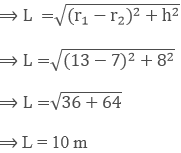Curved surface area of the frustum (s1) = π(r1 + r2) × L =  π(13 + 7) × 10 = 200 π m2

Then, given slant height of conical cap = 12 m

Base radius of upper cap cone = 7 m

So, the curved surface area of upper cap cone (s2) = πrl = π × 7 × 12 = 264 m2

Thus, the total canvas required for tent (S) = s1 + s2

S = 200π + 264 = 892.57 m2

Therefore, the canvas required for the tent is 892.57 m2.

10. A milk container of height 16 cm is made of metal sheet in the form of frustum of a cone with radii of its lower and upper ends as 8 cm and 20 cm respectively. Find the cost of milk at the rate of Rs.44 per litre which the container can hold.

Solution:

Given,

A milk container of the form of frustum of a cone

The radius of the lower end (r1) = 8 cm

The radius of the upper end (r2) = 20 cm

Let h be its height, h = 16 cm

Then, the capacity of the container = Volume of frustum of the cone

= 1/3 π(r22 + r12 + r1 r)h

= 1/3 π(202 + 82 + (20) (8) ) x 16

= 10459.42 cm3

= 10.46 litres

Now, given that cost of 1 litre of milk = Rs 44

Then the cost of 10.46 litres of milk = Rs (44 x 10.46) = Rs 460.24

11. A bucket is in the form of a frustum of a cone of height 30 cm with radii of its lower and upper ends as 10 cm and 20 cm respectively. Find the capacity and surface area of the bucket. Also, find the cost of milk which can completely fill the container, at the rate of Rs.25 per litre.

Solution:

Let R and r be the radii of the top and base of the bucket respectively,

Let h be its height.

Then, we have R = 20 cm, r = 10 cm, h = 30 cm

Capacity of the bucket = Volume of the frustum of the cone

= 1/3 π(R2 + r2 + R r )h

= 1/3 π(202 + 102 + 20 x 10 ) x 30

= 3.14 x 10 (400 + 100 + 200)

= 21980 cm3 = 21.98 litres

Now,

Surface area of the bucket = CSA of the bucket + Surface area of the bottom

= π l (R + r) + πr2

We know that,

l = √h2 + (R – r)2

= √[302 + (20 – 10)2] = √(900 + 100)

= √1000 = 31.62 cm

So,

The Surface area of the bucket = (3.14) x 31.62 x (20 + 10) + (3.14) x 102

= 2978.60 + 314

= 3292.60 cm2

Next, given that the cost of 1 litre milk = Rs 25

Thus, the cost of 21.98 litres of milk = Rs (25 x 21.98) = Rs 549.50